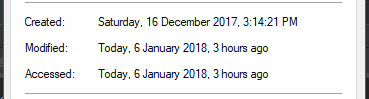# Change File Modification Time In Python

6 Jan 2018 Tutorials pythonThis post demonstrates how to change a file modification time in Python. No third party modules are required and it will work on Windows, Mac and Linux.

## File Modification Times

File modification times show when a file was last edited. This can sometimes be confused with creation time but these are very different. Creation time is normally held by the operating system and states when a file was created. This means if you download a file from the internet, the creation time will change and be the time it was downloaded. Thus the creation time isn't very helpful.

File modification time is different however as it is stored in the file. Even though the operating system still manages these, they can still be easily changed as opposed to creation time.

The modification date can be found by right-clicking on a file and selecting properties.## Setting File Modification Times

First, you will want to import os, time and datetime.

``````import os
import time
import datetime
``````

You will now need to locate the file you want to edit and create a time object to set to the file. To create one, we will first break it down into its simpler parts.

``````fileLocation = r""
year = 2017
month = 11
day = 5
hour = 19
minute = 50
second = 0
``````

fileLocation is a string and the rest of the variables above are integers.

Next, we will create our datetime object using the data given and then convert it to seconds since epoch; this is what will be stored.

``````date = datetime.datetime(year=year, month=month, day=day, hour=hour, minute=minute, second=second)
modTime = time.mktime(date.timetuple())
``````

Now we can do a simple os.utime call passing the file and modification time to set the new times.

``````os.utime(fileLocation, (modTime, modTime))
``````

Now if you go back and check the modification date it should be changed.

## Final Code

``````import os
import time
import datetime

fileLocation = r""
year = 2017
month = 11
day = 5
hour = 19
minute = 50
second = 0

date = datetime.datetime(year=year, month=month, day=day, hour=hour, minute=minute, second=second)
modTime = time.mktime(date.timetuple())

os.utime(fileLocation, (modTime, modTime))
``````

## FAQ

### But how do I change creation time?

The solution is platform-specific but for Windows you can look at this.